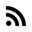# Details of C that every noob forgets

I am still forgetting some of them, that’s why I wrote this post ;)

# C Details

## Floating numbers

The IEEE 754 standard specifies that a floating-point number will be represented as follows:

• Sign bit: 1 bit
• Exponent width: 8 bits
• Significant precision: 23 bits

And a double-precision floating-point number:

• Sign bit: 1 bit
• Exponent width: 11 bits
• Significant precision: 52 bits

The `%` operator cannot be applied to a float or double.

## Parenthesis counts

`!=` has a higher “range” or precedence than “=”, which means that:

``````c = getchar() != EOF
``````

is equivalent to

``````c = (getchar() != EOF)
``````

`*p++` is not the same as `(*p)++`. In the first case `p++` is performed first. Read more about C Operator Precedence.

In general, use parenthesis and/or split statements when they could be confusing such as:

``````int a, b=2, c=3;
a=b=c;
printf("a=%d\tb=%d\tc=%d\n",a,b,c);

/* Output */
a=3	b=3	c=3
``````

It is better write that code as:

``````int a, b=2, c=3;
b=c;
a=b;

``````

## Be careful with the quotes

• `'a'` is not the same as `"a"`. `'a'` is a `char` with the numeric value of `97 (0x61)`, whereas `"a"` is an `char` array defined as `[ 'a', '\0']`. Where `'\0'` is the null character.

## Please, give me a soul

• `extern` and `static` variables are initialized to zero by default. Automatic and `register` variables will have an undefined values if they are not initialized.

• `malloc()` does not initialize the allocated memory, `calloc()` does.

## Assignment operators

Do not forget that:
`x *= y+a` is the same as `x = x * ( y+a )`

## C gives a shit about indentation

In case of possible ambiguity it is better to use braces to avoid bugs.

``````/* Clear case:*/
if ( n>9 )
n++;
else
n--;
``````

In the code above, the compiler will have no problem in understand what you want to do, but is the following case, there will be same problems even though it is “clear” for a human to understand what you want to do. It seems that `else` belongs to the outer `if` but, in reality, it does not.

``````/* Case with ambiguity*/
if ( n>9 )
for ( i=0 ; i<3; i++ )
if ( i*4 > n )
i++;
else
n--;
``````

Thus, use braces as follows:

``````/* Case with ambiguity*/
if ( n>9 ){
for ( i=0 ; i<3; i++ )
if ( i*4 > n )
i++;
}
else
n--;
``````

## My mind is here but my body somewhere else

Do not forget the keyword `extern` when you are going to use a variable that is it defined in some other file.

``````/* file 1*/
extern int f[];

void main(int x){
f = x;
}

/* file 2 */
int f[] = {4,5,6};
``````

Of course, do not forget to link that file to your source. For example `gcc -o a.out file1.c file2.c`.

## Breaks

DO NOT FORGET that `break` statement does NOT provide an early exit from `if`, but it does from `for`, `while`, `do` and `switch`.

## Strings and commas

Be careful with the commas when defining `char` arrays, or working with strings in general.

``````char *words[] = {
"hi",
/*no comma*/
"hello"
"danke",
"hola"
}
``````

If we print each element of that array, we will get this:

``````    "hi"
"hellodanke"
"hola"
``````

Since C concatenates strings the program printed `"hellodanke"`.

# Recommendations

## Use keyword to keep your code safe

• Cast `signed` or `unsigned` if the operand will be have either positive and negative values, or non-negative values. So the compiler doesn’t have to choose.
``````int i =-1;
unsigned int j = 2;

/* don't forget that
i = 0xFFFF
j = 0x2   */
if ( i < j)
puts("i < j");
else
puts("actually, i > j");
``````

The above code prints `"actually, i > j`, since `j` is `unsigned` so `i` is also treated as `unsigned`. Thus, `0xffff > 0x2`. You should cast `j` as `signed` to make the condition true.

``````if ( i < (signed) j)
puts("i < j");
else
puts("actually, i > j");
``````
• Use `const` if you don’t want the variable to change (read-only status), or it shouldn’t be modified in a function.
• Use `static` if you want to hide variables or functions declarations inside modules. It is similar to `private` keyword in c++;

## Use `void` due to backwards compatibility

`void` states explicitly as empty list. Read more here

The code below works but there is no verification. `test` is not supposed to return anything, but due to backwards compatibility `test` could be defined as if it returned something. And this my friend is a bug.

``````/*  old C style  */
/* declaration */
test( int );

int main(){
test(5);
return 0;
}

/* definition */
test( input1 )
int input1;
{
return (input1 + 4);
}
``````

## Last words

That’s all I have in mind for this moment. I hope this post helped you as much as it helped me. I will update this post if I remember more mistakes or if you send me more.

Cheers :3